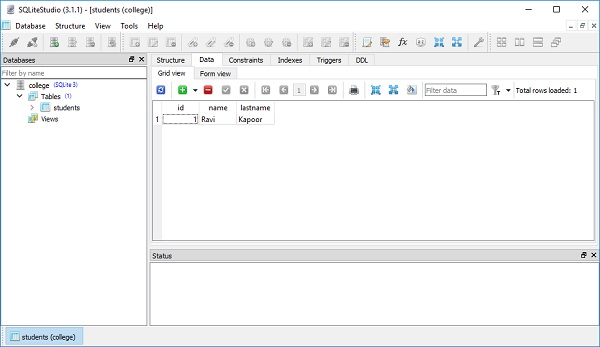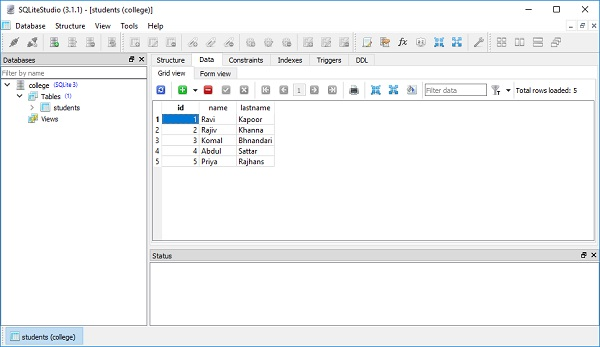# SQLAlchemy Core - Executing Expression

In the previous chapter, we have learnt SQL Expressions. In this chapter, we shall look into the execution of these expressions.

In order to execute the resulting SQL expressions, we have to obtain a connection object representing an actively checked out DBAPI connection resource and then feed the expression object as shown in the code below.

```conn = engine.connect()
```

The following insert() object can be used for execute() method −

```ins = students.insert().values(name = 'Ravi', lastname = 'Kapoor')
result = conn.execute(ins)
```

The console shows the result of execution of SQL expression as below −

```INSERT INTO students (name, lastname) VALUES (?, ?)
('Ravi', 'Kapoor')
COMMIT
```

Following is the entire snippet that shows the execution of INSERT query using SQLAlchemy’s core technique −

```from sqlalchemy import create_engine, MetaData, Table, Column, Integer, String
engine = create_engine('sqlite:///college.db', echo = True)

students = Table(
'students', meta,
Column('id', Integer, primary_key = True),
Column('name', String),
Column('lastname', String),
)

ins = students.insert()
ins = students.insert().values(name = 'Ravi', lastname = 'Kapoor')
conn = engine.connect()
result = conn.execute(ins)
```

The result can be verified by opening the database using SQLite Studio as shown in the below screenshot −The result variable is known as a ResultProxy object. It is analogous to the DBAPI cursor object. We can acquire information about the primary key values which were generated from our statement using ResultProxy.inserted_primary_key as shown below −

```result.inserted_primary_key

```

To issue many inserts using DBAPI’s execute many() method, we can send in a list of dictionaries each containing a distinct set of parameters to be inserted.

```conn.execute(students.insert(), [
{'name':'Rajiv', 'lastname' : 'Khanna'},
{'name':'Komal','lastname' : 'Bhandari'},
{'name':'Abdul','lastname' : 'Sattar'},
{'name':'Priya','lastname' : 'Rajhans'},
])
```

This is reflected in the data view of the table as shown in the following figure −